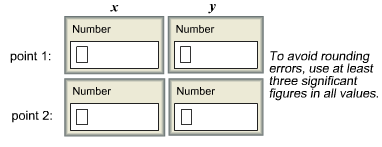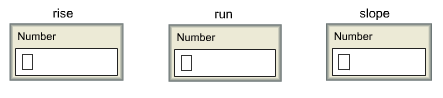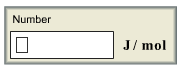# Problem: Consider this reaction data:A -&gt; productsT(K)    k(s-1)325     0.380725     0.868If you were going to graphically determine the activation energy of this reaction, what points would you plot?Determine the rise, run, and slope of the line formed by these pointsWhat is the activation energy of this reaction?

###### FREE Expert Solution

We need to use the linearized Arrhenius Equation:

where:

k = rate constant

Ea = activation energy (in J/mol)

R = gas constant (8.314 J/mol • K)

T = temperature (in K)

A = Arrhenius constant or frequency factor

82% (398 ratings)###### Problem Details

Consider this reaction data:
A -> products
T(K)    k(s-1)
325     0.380
725     0.868

If you were going to graphically determine the activation energy of this reaction, what points would you plot?Determine the rise, run, and slope of the line formed by these pointsWhat is the activation energy of this reaction?Frequently Asked Questions

What scientific concept do you need to know in order to solve this problem?

Our tutors have indicated that to solve this problem you will need to apply the Arrhenius Equation concept. You can view video lessons to learn Arrhenius Equation. Or if you need more Arrhenius Equation practice, you can also practice Arrhenius Equation practice problems.